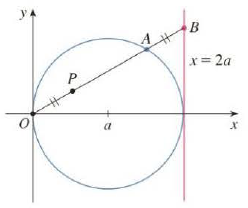Chapter 10.1, Problem 44E

Chapter
Section
Textbook Problem

# (a) Find parametric equations for the set of all points P as shown in the figure such that |OP| = |AB|. (This curve is called the cissoid of Diodes after the Greek scholar Diodes, who introduced the cissoid as a graphical method for constructing the edge of a cube whose volume is twice that of a given cube.) (b) Use the geometric description of the curve to draw a rough sketch of the curve by hand. Check your work by using the parametric equations to graph the curve.(a)

To determine

To find: The parametric equations. of all the set of points P .

Explanation

Given:

The curve Cissoid of Diocles is shown in below figure1.

Calculation:

Let θ be the angle of inclination of segment OP .

OB=2acosθ

The point of C be (2a,0) .

The right triangle OAC .

OA=2acosθ

OP=ABOP=OBOA

Substitute (2acosθ) for |OA| and (2acosθ) for |OB| in above equation

(b)

To determine

To plot: The curve using the parametric equations. x=2asin2θ and y=2asin2θtanθ .

### Still sussing out bartleby?

Check out a sample textbook solution.

See a sample solution

#### The Solution to Your Study Problems

Bartleby provides explanations to thousands of textbook problems written by our experts, many with advanced degrees!

Get Started

#### In Exercises 516, evaluate the given quantity. log416

Finite Mathematics and Applied Calculus (MindTap Course List)

#### In problems 49-54, rationalize the denominator and then simpify. 51.

Mathematical Applications for the Management, Life, and Social Sciences

#### Evaluate the integral. 31. 1x31dx

Single Variable Calculus: Early Transcendentals

#### True or False: xy + 6x2y = 10 x3 is linear.

Study Guide for Stewart's Single Variable Calculus: Early Transcendentals, 8th